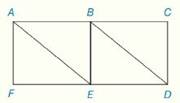Chapter 4.CR, Problem 26CRElementary Geometry For College St...

7th Edition
Alexander + 2 others
ISBN: 9781337614085

Solutions

Chapter
SectionElementary Geometry For College St...

7th Edition
Alexander + 2 others
ISBN: 9781337614085
Textbook Problem

Review Exercises Given: A B E F is a rectangle B C D E is a rectangle F E - = E D - Prove: A E - ≅ B D -   and   A E - ∥ B D -To determine

To Prove:

For the provided rectangles ABEF and BCDE, AE-BD- and AE-BD- with the help of the given information

Explanation

Proof:

Theorem on parallel lines:

If two lines are cut by a transversal line and the alternate exterior angles are congruent, the lines are parallel.

Description:

It is given that ABEF and BCDE are rectangle attached to each other as shown in the picture with FE-=ED-.

Since FE-=ED-, rectangles ABEF and BCDE have common base and height.

Hence the rectangle ABEF and the rectangle BCDE are congruent.

Since the rectangles are congruent, their diagonals must be same.

This implies AE-BD-.

Considering the line BE as a transversal line for the lines AE and BD with its same alternate interior angles (mDBE=mBEA), it can conclude from the theorem that these lines are parallel to each other

Still sussing out bartleby?

Check out a sample textbook solution.

See a sample solution

The Solution to Your Study Problems

Bartleby provides explanations to thousands of textbook problems written by our experts, many with advanced degrees!

Get Started

In Exercises 4562, find the values of x that satisfy the inequality (inequalities). 48. 12 3x

Applied Calculus for the Managerial, Life, and Social Sciences: A Brief Approach

Explain why the formula for sample variance is different from the formula for population variance.

Essentials of Statistics for The Behavioral Sciences (MindTap Course List)

4. Compute .

Mathematical Applications for the Management, Life, and Social Sciences

Sometimes, Always, or Never: The sum of two elementary functions is an elementary function.

Study Guide for Stewart's Single Variable Calculus: Early Transcendentals, 8th

For , f′(x) =

Study Guide for Stewart's Multivariable Calculus, 8th

What are the five steps of the scientific method?

Research Methods for the Behavioral Sciences (MindTap Course List)

Complete each factoring formula. x2+2xy+y2=.

College Algebra (MindTap Course List)